Test your math knowledge in this quiz on congruence.

# Congruence

This Math quiz is called 'Congruence' and it has been written by teachers to help you if you are studying the subject at high school. Playing educational quizzes is a user-friendly way to learn if you are in the 9th or 10th grade - aged 14 to 16.

It costs only \$12.50 per month to play this quiz and over 3,500 others that help you with your school work. You can subscribe on the page at Join Us

This high school Math quiz is all about Congruence – one of those words that has meaning in math and nowhere else!

Two shapes are congruent if they are identical in every way – all corresponding sides are the same length, and all corresponding angles are identical. If you have tried the Similarity (F) quiz you might recognize some… well, similarities. Recall that two shapes are similar if their corresponding angles are equal, and corresponding sides are in the same ratio.

Shapes can be congruent to each other even if one of them has been reflected or rotated, so don’t get caught out by that little trick!

Triangles are a common shape to come up in questions on congruency, and there are 4 different ways to show it. You need to be familiar with each of them.

ASA – Angle, Side, Angle. Show that 2 angles in each triangle are the same, and a corresponding side is the same length.

SAS – Side, included Angle, Side. Show that 2 pairs of corresponding sides are equal, and the angle formed between these 2 sides.

SSS – Side, Side, Side. Show that all 3 pairs of corresponding sides are equal.

RHS – Right-angle, Hypotenuse, Side. This is a special case for right triangles. Show that the hypotenuse and one other side of each triangle is the same.

Remember these three-letter abbreviations, as you can state them as your reasoning when it comes to the exam.

Question 1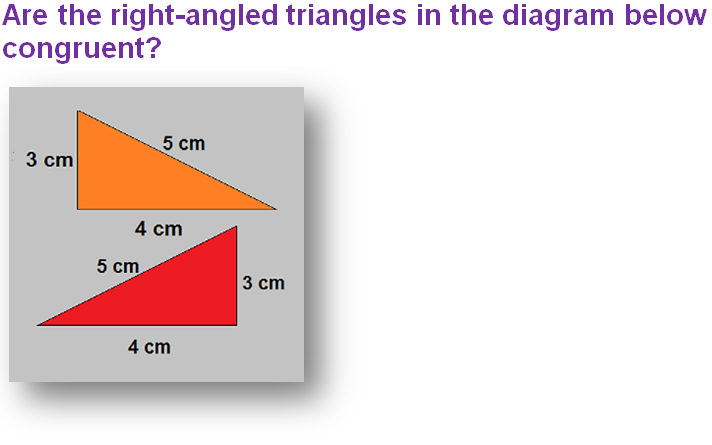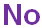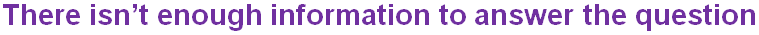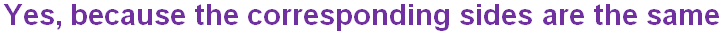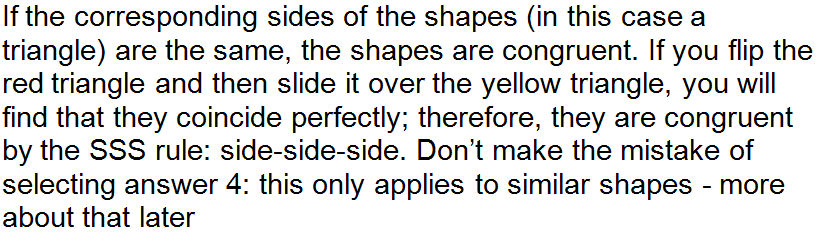Question 2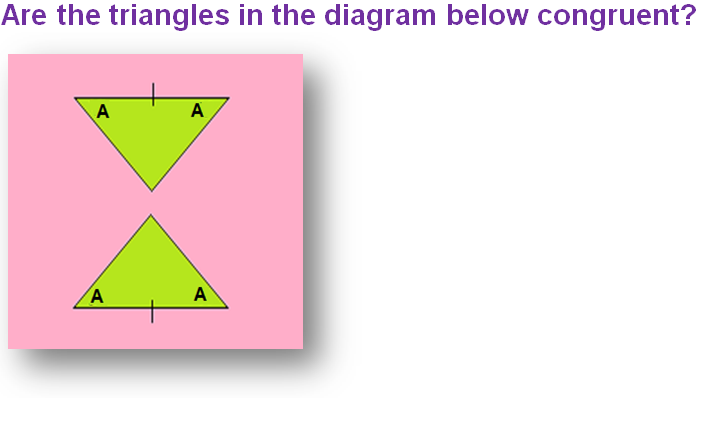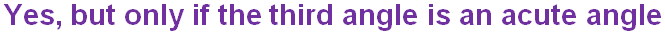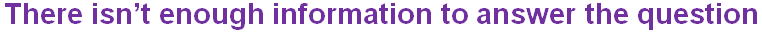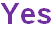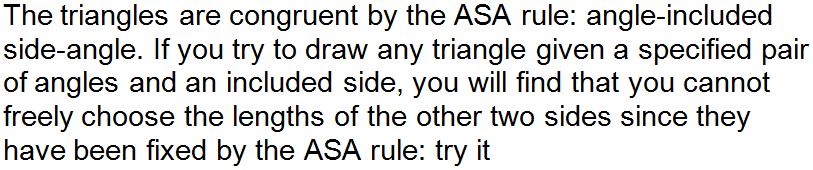Question 3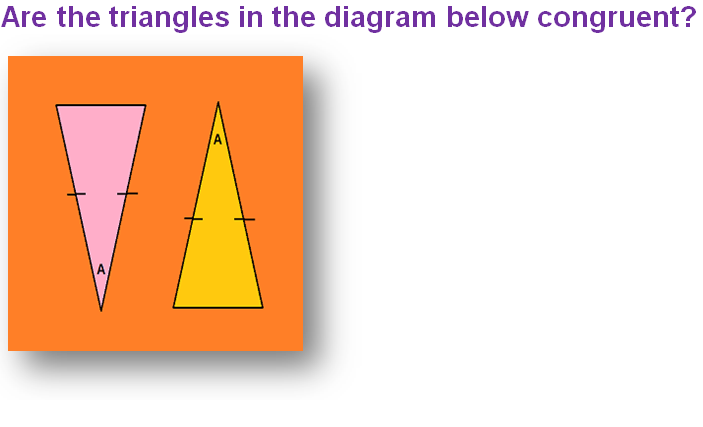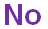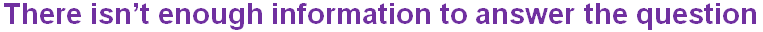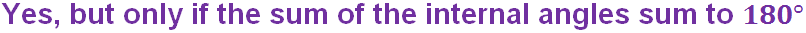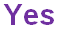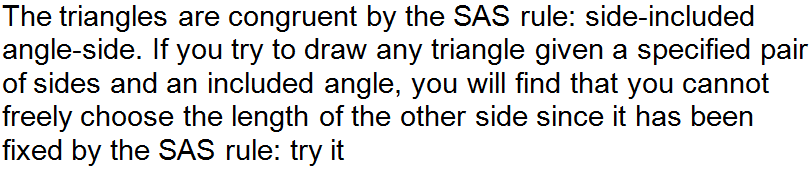Question 4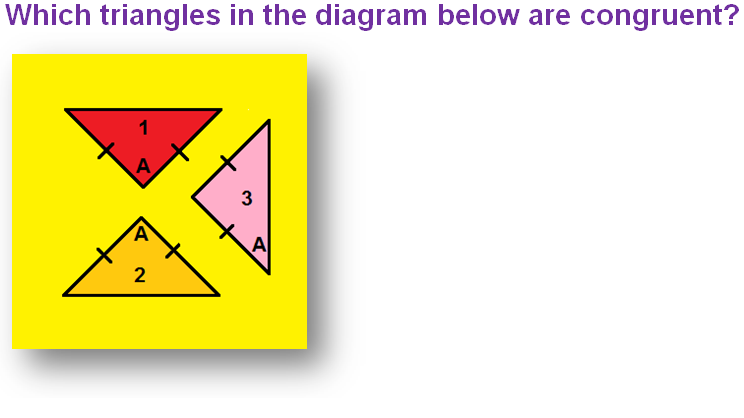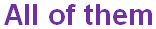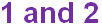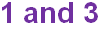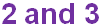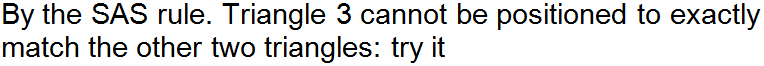Question 5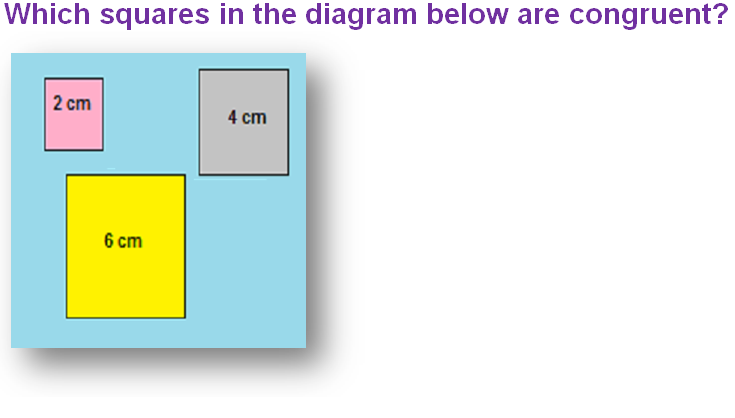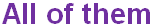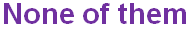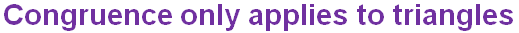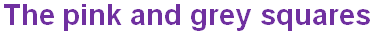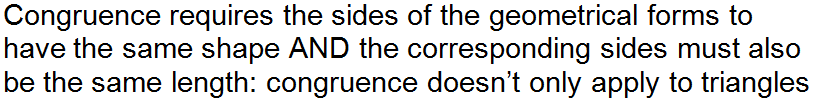Question 6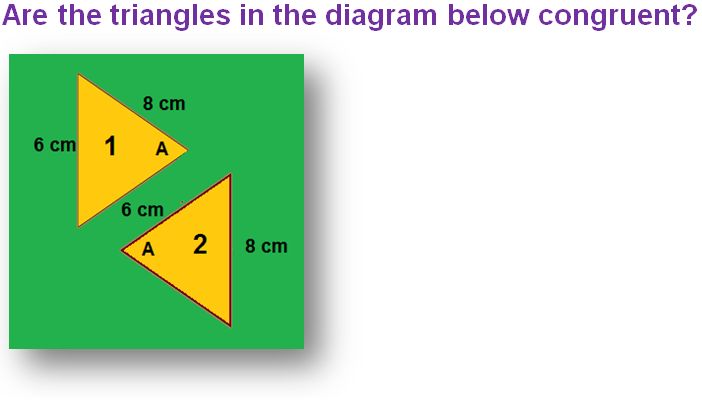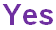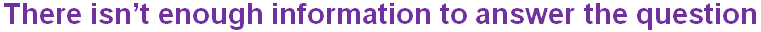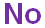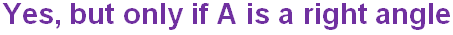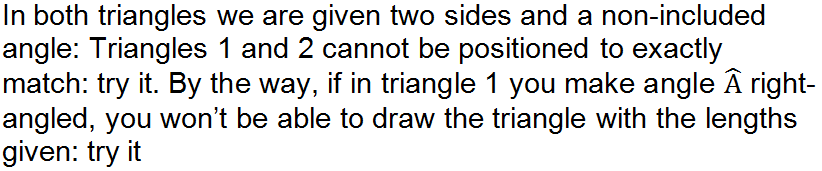Question 7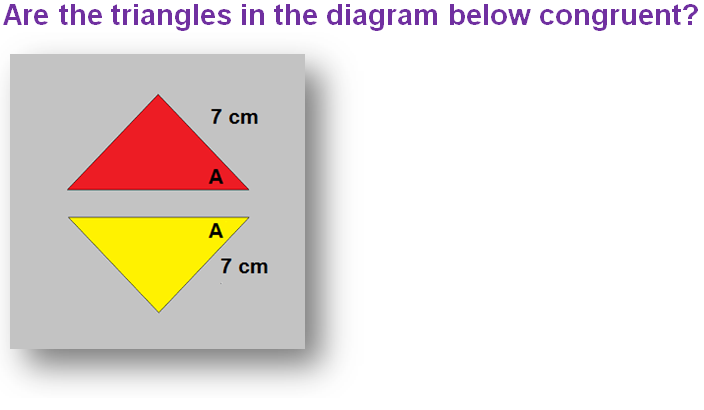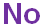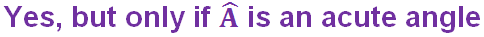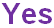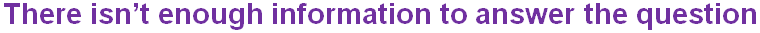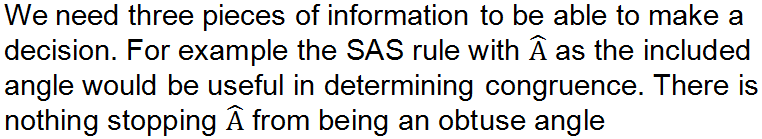Question 8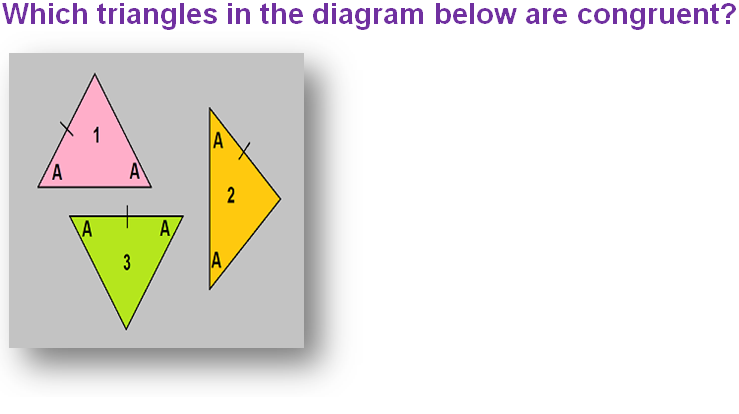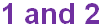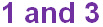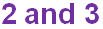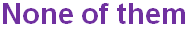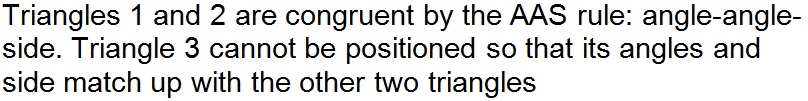Question 9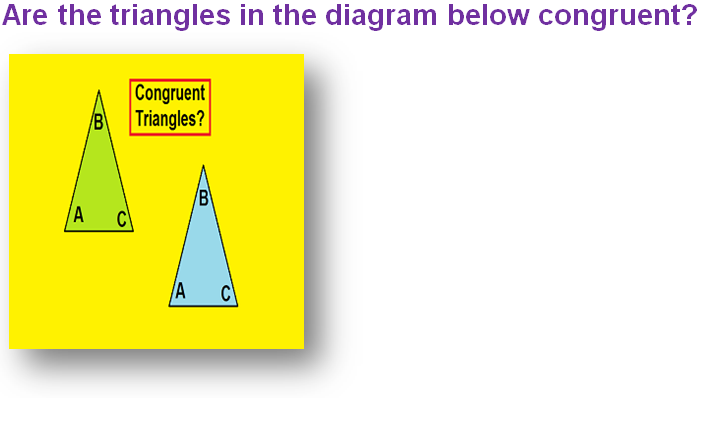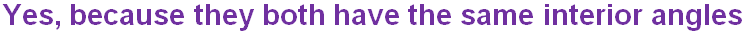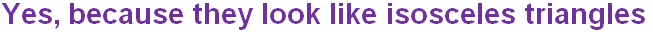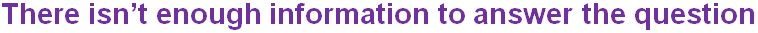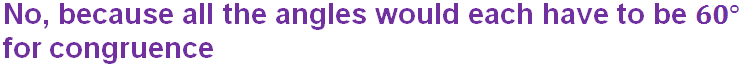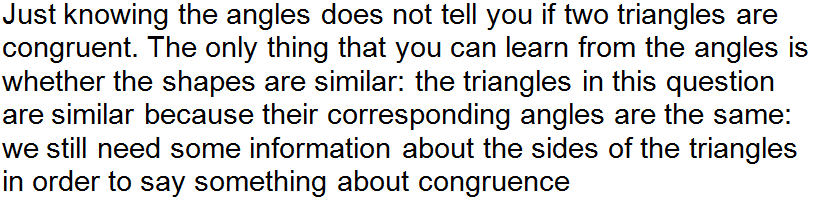Question 10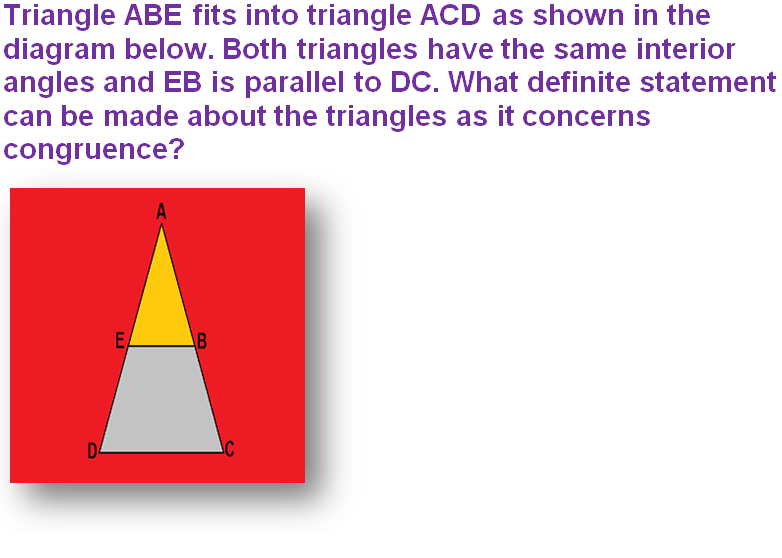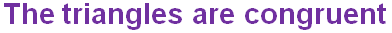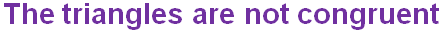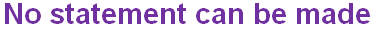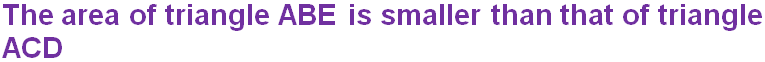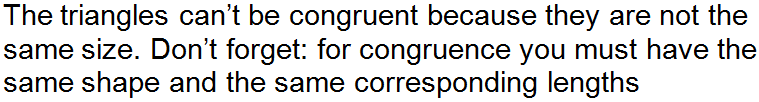Author:  Frank Evans